Question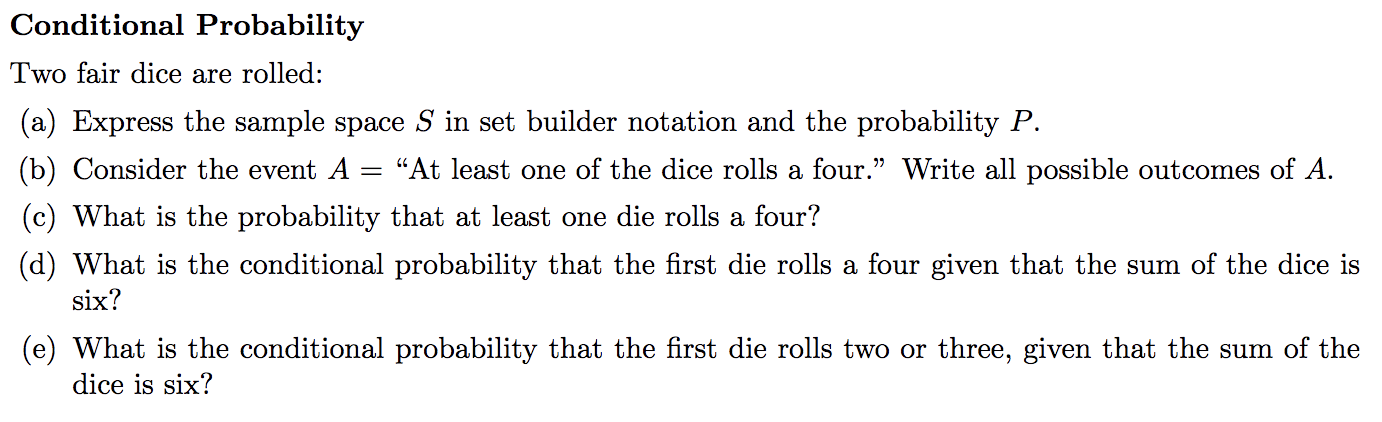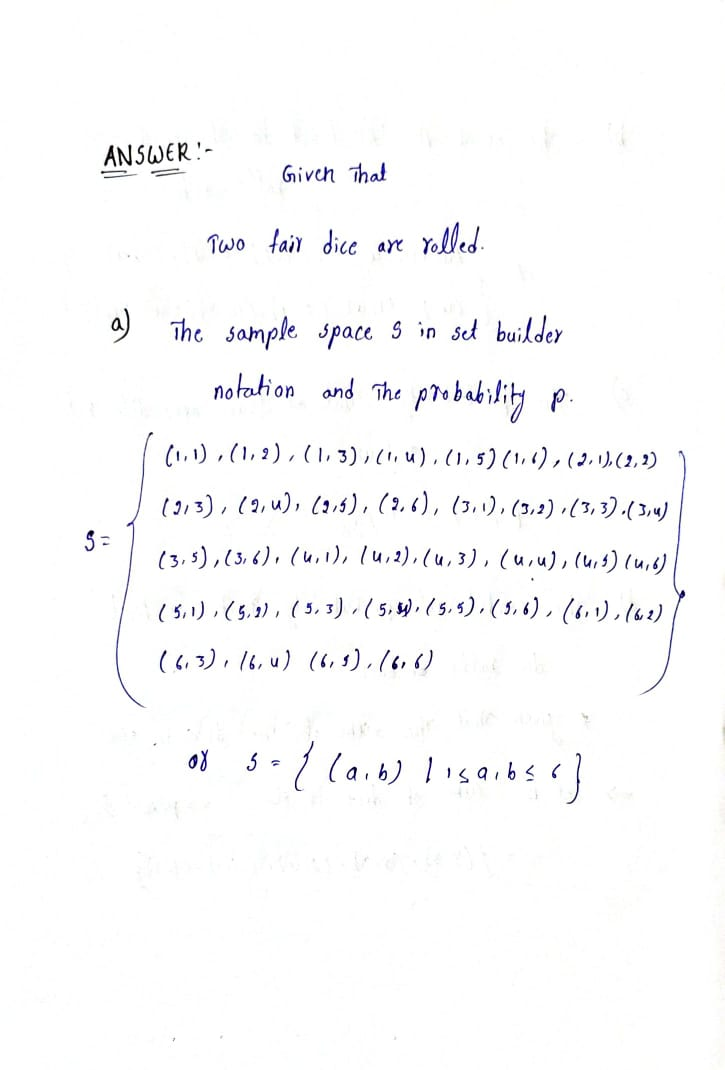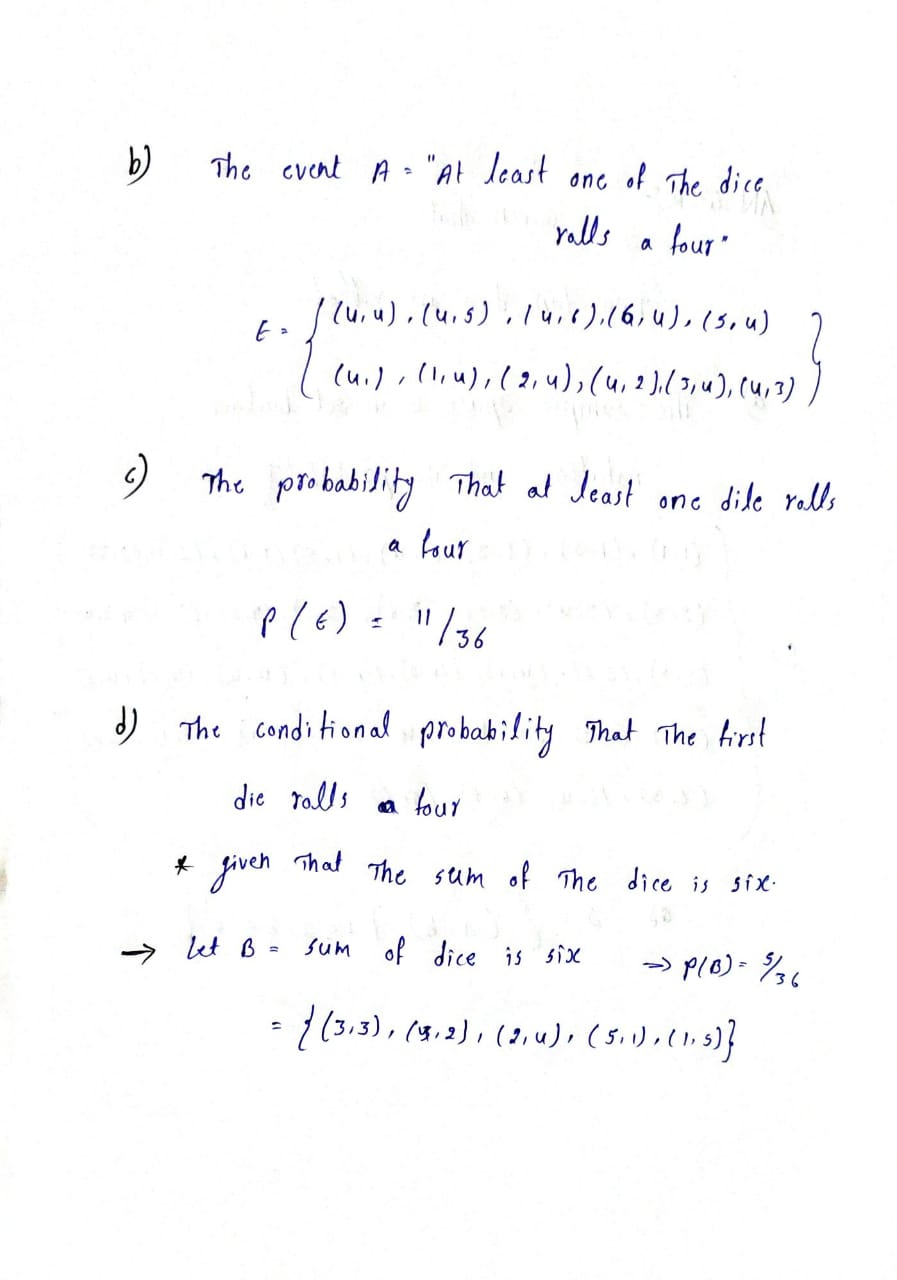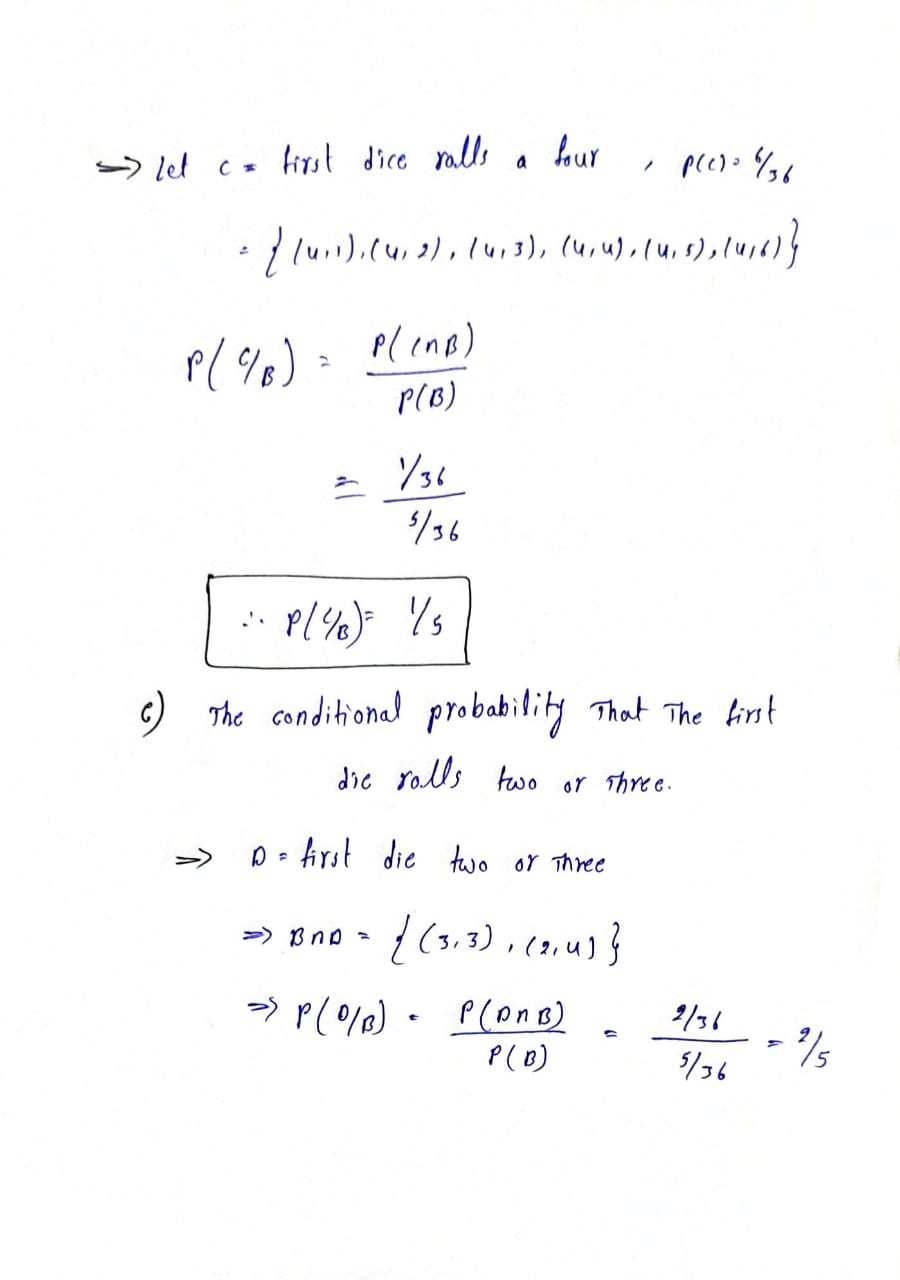#### Earn Coins

Coins can be redeemed for fabulous gifts.

Similar Homework Help Questions
• ### 1. Find the conditional probability of the indicated event when two fair dice (one red and...

1. Find the conditional probability of the indicated event when two fair dice (one red and one green) are rolled. The sum is 4, given that the green one is either 2 or 3. Find the conditional probability of the indicated event when two fair dice (one red and one green) are rolled. The sum is 10, given that the dice have opposite parity.

• ### Two dice are rolled one after the other. Construct a sample space and determine the probability...

Two dice are rolled one after the other. Construct a sample space and determine the probability that the sum of the dots on the dice total 9 if the first die is a 4 . The probability that the sum of the dots on the dice total 9 if the first die is a 4 is one sixth .

• ### Two fair dice are rolled. Let A be the event the sum is even and B...

Two fair dice are rolled. Let A be the event the sum is even and B be the event at least one of the numbers rolled is three. (a) What is the sample space? (b) Display the outcomes in a Karnaugh map in terms of events A and B. (c) Determine P(AB).

• ### If two fair dice are rolled, what is the conditional probability that the rst one lands...

If two fair dice are rolled, what is the conditional probability that the rst one lands on an even number given that the second one lands on a number less than or equal to four? Compute the conditional probability that the second one lands on a number less than four given that the rst one lands on an even number.

• ### Find the conditional probability. Round to 3 decimal places. If two fair dice are rolled, find...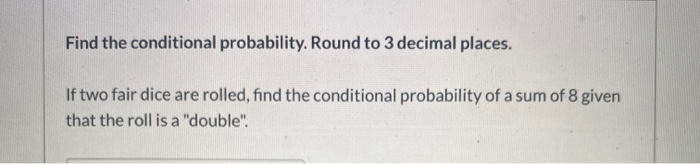Find the conditional probability. Round to 3 decimal places. If two fair dice are rolled, find the conditional probability of a sum of 8 given that the roll is a "double".

• ### Rolling Dice 2. A pair of dice is rolled. Here is the sample space (all of...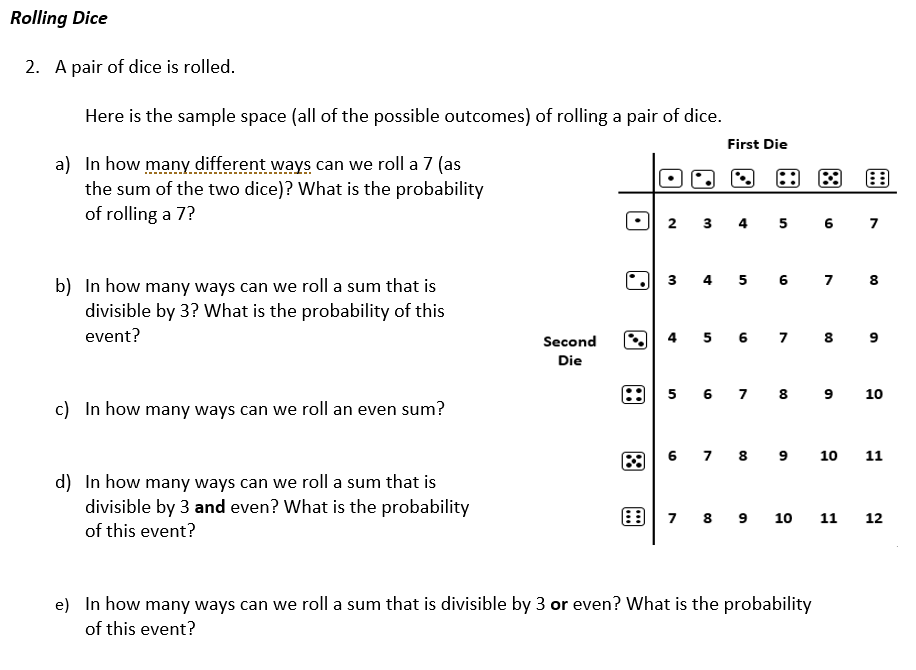Rolling Dice 2. A pair of dice is rolled. Here is the sample space (all of the possible outcomes) of rolling a pair of dice. First Die a) In how many different ways can we roll a 7 (as the sum of the two dice)? What is the probability of rolling a 7? 2 3 4 5 6 7 3 4 5 6 7 8 b) In how many ways can we roll a sum that is divisible by 3?...

• ### Problem 1 (10 points). If two fair dice are rolled 10 times, what is the probability...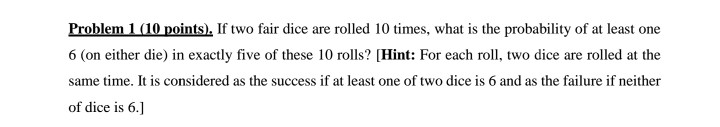Problem 1 (10 points). If two fair dice are rolled 10 times, what is the probability of at least one 6 (on either die) in exactly five of these 10 rolls? (Hint: For each roll, two dice are rolled at the same time. It is considered as the success if at least one of two dice is 6 and as the failure if neither of dice is 6.]

• ### 2. Roll two fair dice. (a) How many possible outcomes are there? (b) What probability would...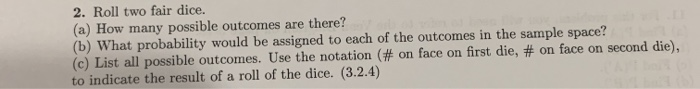2. Roll two fair dice. (a) How many possible outcomes are there? (b) What probability would be assigned to each of the outcomes in the sample space? (c) List all possible outcomes. Use the notation (# on face on first die, # on face on second die), to indicate the result of a roll of the dice. (3.2.4)

• ### Abdul rolls a fair six-sided die and a fair four-sided die simultaneously. The sample space of...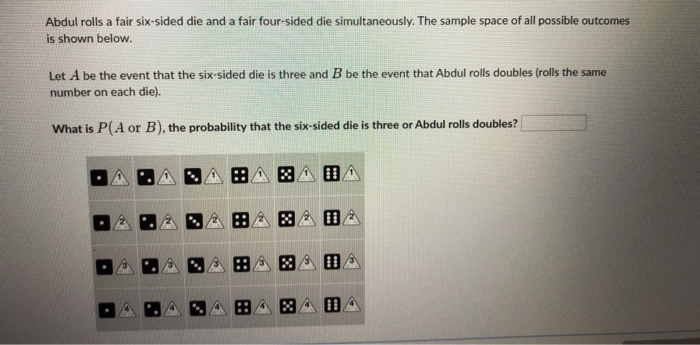Abdul rolls a fair six-sided die and a fair four-sided die simultaneously. The sample space of all possible outcomes is shown below. Let A be the event that the six-sided die is three and B be the event that Abdul rolls doubles (rolls the same number on each die). What is PCA or B), the probability that the six-sided die is three or Abdul rolls doubles? DAOANAA BADA 02. \$ 2 82 02

• ### 5. What is the correct set notation for the event that "the sum of the two dice is not less than 5 if at...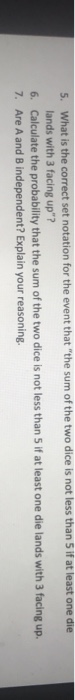5. What is the correct set notation for the event that "the sum of the two dice is not less than 5 if at least one die lands with 3 facing up"? 6. Calculate the probability that the sum of the two dice is not less than 5 if at least one die lands with 3 facing up. 7. Are A and B independent? Explain your reasoning. Use for Questions 1-7: Hector will roll two fair, six-sided dice at the...